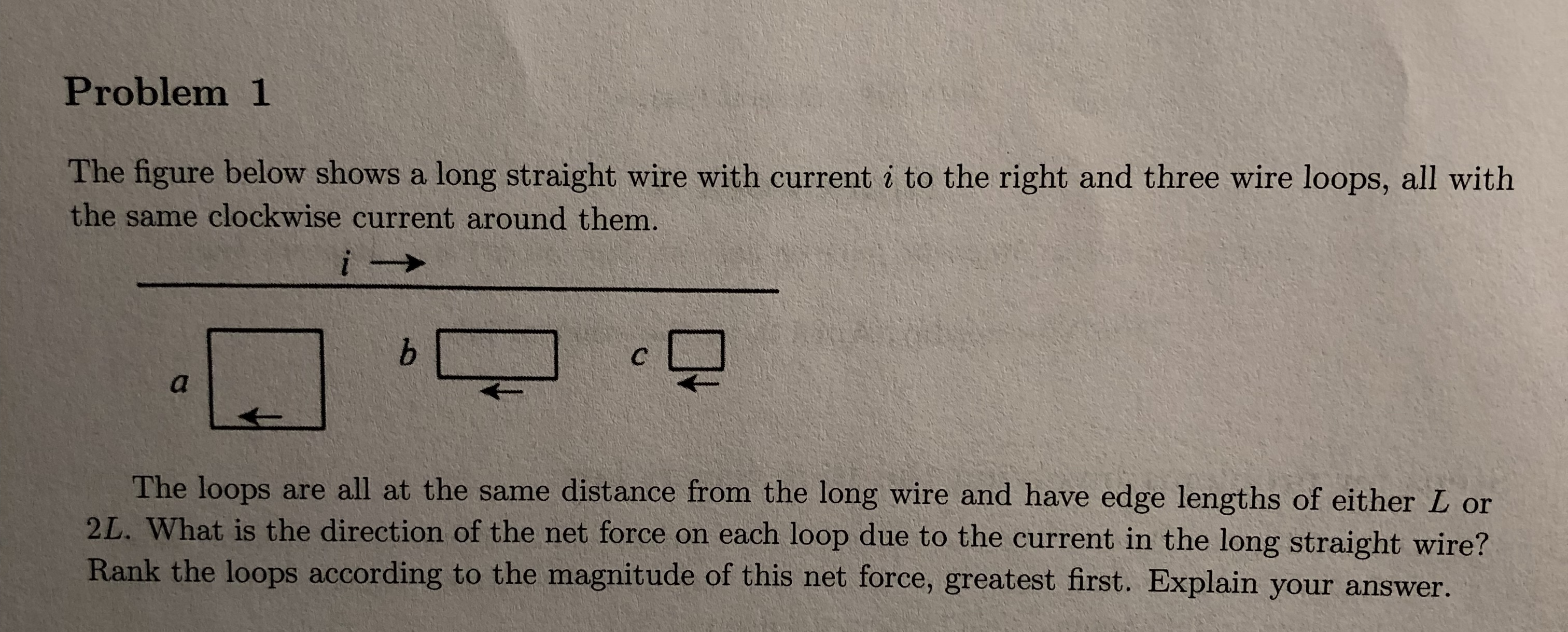Problem 1The figure below shows a long straight wire with current i to the right and three wire loops, all withthe same clockwise current around them.The loops are all at the same distance from the long wire and have edge lengths of either L or2L. What is the direction of the net force on each loop due to the current in the long straight wire?Rank the loops according to the magnitude of this net force, greatest first. Explain your answer.

Questionhelp_outlineImage TranscriptioncloseProblem 1 The figure below shows a long straight wire with current i to the right and three wire loops, all with the same clockwise current around them. The loops are all at the same distance from the long wire and have edge lengths of either L or 2L. What is the direction of the net force on each loop due to the current in the long straight wire? Rank the loops according to the magnitude of this net force, greatest first. Explain your answer. fullscreen
Step 1

The diagram for the loops is given below:

Step 2

If the direction of the current through the loop and the current carrying wire is in same direction, the magnetic force is more attractive than when the direction of the current through the loop and the current carrying wire is in opposite direction.

Thus, the for...

Want to see the full answer?

See Solution

Want to see this answer and more?

Our solutions are written by experts, many with advanced degrees, and available 24/7

See Solution
Tagged in

Physics